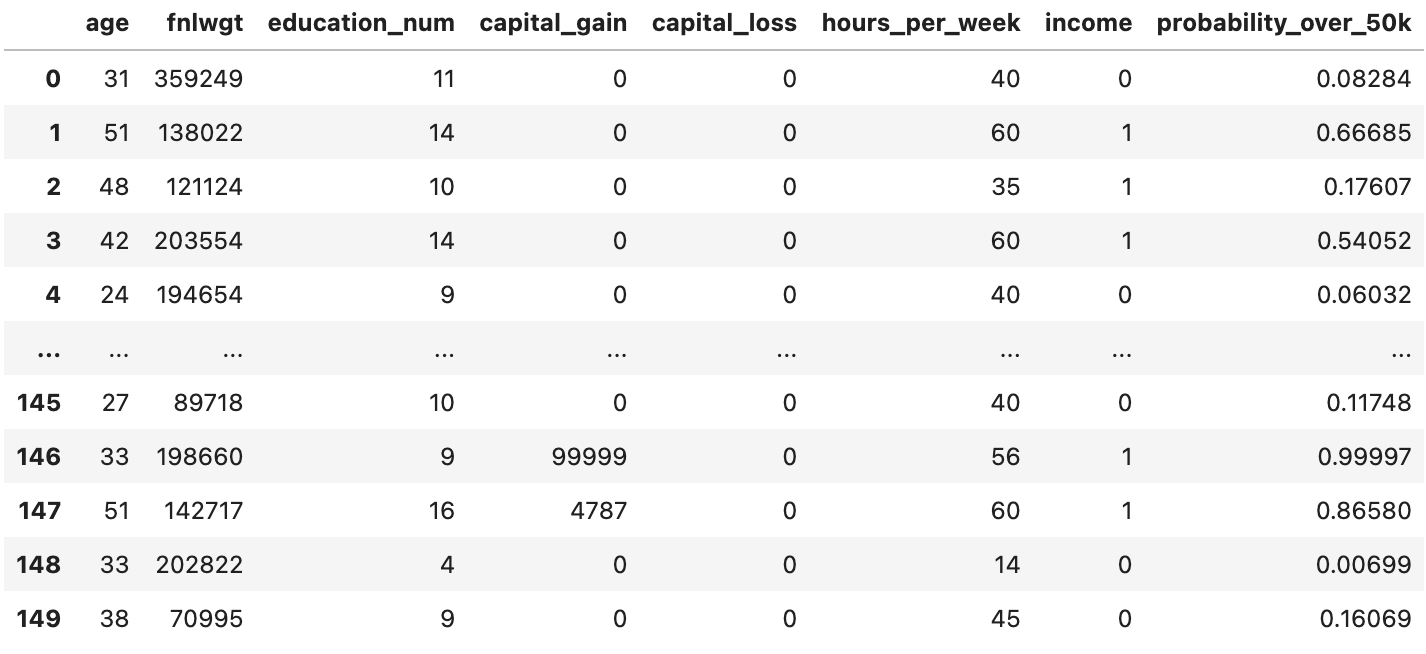# Registering a Binary Classification Model

Suppose you would like to register a binary classification model for the following dataset.Following is an example of how you would construct a `fdl.ModelInfo` object and register such a model.

``````PROJECT_ID = 'example_project'
MODEL_ID = 'binary_model'

dataset_info = client.get_dataset_info(
project_id=PROJECT_ID,
dataset_id=DATASET_ID
)

model_target = 'income'
model_outputs = ['probability_over_50k']
model_features = [
'age',
'fnlwgt',
'education_num',
'capital_gain',
'capital_loss',
'hours_per_week'
]

model_info = fdl.ModelInfo.from_dataset_info(
dataset_info=dataset_info,
dataset_id=DATASET_ID,
target=model_target,
outputs=model_outputs,